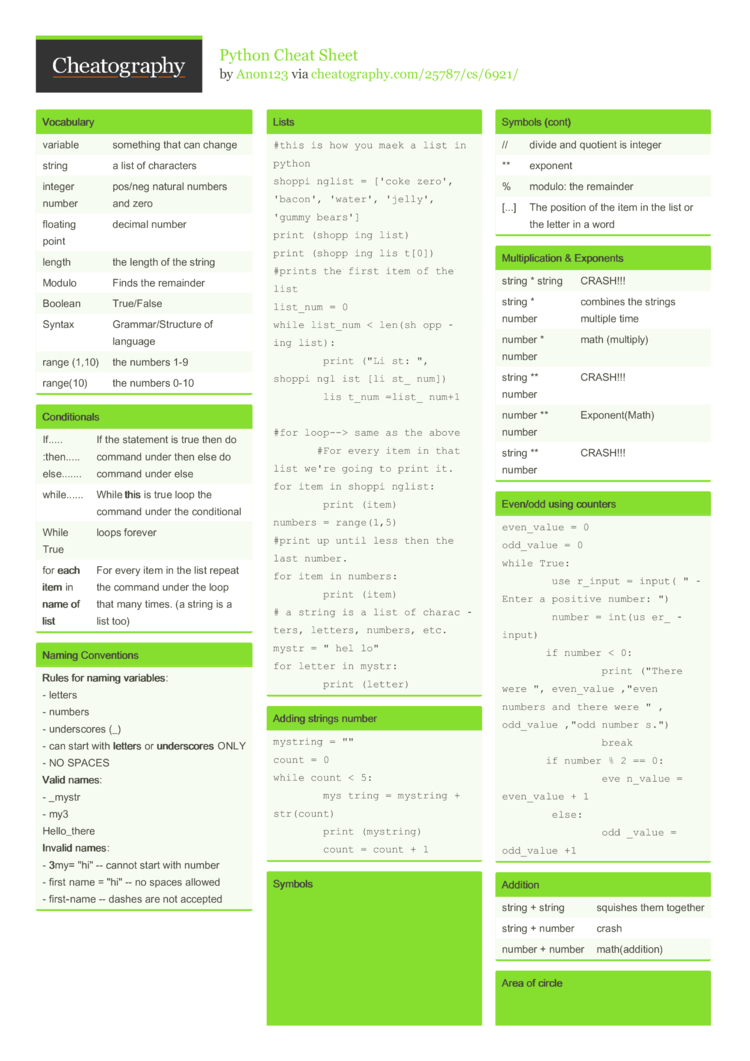# Python Cheat Sheet by Anon123

Cheat Sheet

### Vocabulary

 variable something that can change string a list of characters integer number pos/neg natural numbers and zero floating point decimal number length the length of the string Modulo Finds the remainder Boolean True/False Syntax Gramma­r/S­tru­cture of language range (1,10) the numbers 1-9 range(10) the numbers 0-10

### Condit­ionals

 If..... :then..... else....... If the statement is true then do command under then else do command under else while...... While this is true loop the command under the condit­ional While True loops forever for each item in name of list For every item in the list repeat the command under the loop that many times. (a string is a list too)

### Naming Conven­tions

 Rules for naming variab­les: - letters - numbers - unders­cores (_) - can start with lett­ers or unde­rsc­ores ONLY - NO SPACES Valid names: - _mystr - my3 Hello_­there Invalid names: - 3my= "­hi" -- cannot start with number - first name = "­hi" -- no spaces allowed - first­-­name -- dashes are not accepted

### Lists

 ```#this is how you maek a list in python shoppinglist = ['coke zero', 'bacon', 'water', 'jelly', 'gummy bears'] print (shoppinglist) print (shoppinglist) #prints the first item of the list list_num = 0 while list_num < len(shoppinglist):     print ("List:", shoppinglist[list_num])     list_num =list_num+1      #for loop--> same as the above     #For every item in that list we're going to print it. for item in shoppinglist:     print (item) numbers = range(1,5) #print up until less then the last number. for item in numbers:     print (item) # a string is a list of characters, letters, numbers, etc. mystr = "hello" for letter in mystr:     print (letter)```

 ```mystring = "" count = 0 while count < 5:     mystring = mystring + str(count)     print (mystring)     count = count + 1```

### Symbols

 == equal to != not equal to < less than <= less than or equal to > greater than >= greater than or equal to + add - subtract * multiply / divide and quotient is float // divide and quotient is integer ** exponent % modulo: the remainder [...] The position of the item in the list or the letter in a word

### Multip­lic­ation & Exponents

 string * string CRASH!!! string * number combines the strings multiple time number * number math (multiply) string ** number CRASH!!! number ** number Expone­nt(­Math) string ** number CRASH!!!

### Even/odd using counters

 ```even_value = 0 odd_value = 0 while True:     user_input = input("Enter a positive number: ")     number = int(user_input)     if number < 0:         print ("There were ", even_value ,"even numbers and there were " , odd_value ,"odd numbers.")         break     if number % 2 == 0:         even_value = even_value + 1     else:         odd_value = odd_value +1```

 string + string squishes them together string + number crash number + number math(a­ddi­tion)

### Area of circle

 ```def areaofCircle(r):     if r <= 0:         return "Error: invalid radius"          pi = 3.1415     area = pi(r*2)     return (area) user_radius = input('Enter the radius: ') radius = float(user_radius) print("The area of the circle is", areaofCircle(radius))```

### fibonacci

 ```num1= 0 num2=1 mystr = '0' while num1 + num2 < 89:     Fibonacci = num1 +num2          num1= num2          num2=Fibonacci     mystr= mystr+"," + str(num1) print (mystr)```

### Functions

 ```def calc(num1, num2, operation):          if operation == "sum":         return sum(num1, num2)     elif operation == "product":         return product(num1, num2)     elif operation == "diff":         return diff(num1, num2)     elif operation == "div":         return div(num1, num2)      def sum(a, b):     return a+b def product(a, b):     return a*b      def diff (a, b):     return a-b           def div(a, b):     if b == 0:         return ("Error")     elif b != 0:         return a/b           print (calc(1,2,"sum")) print (calc(4,2, "diff")) print (calc (9,0, "div")) print (calc (2,12, "product")) calc(1, 2, "sum")```

### Functions

 print() displays inform­ation on the screen input() receives info from the user int() converts the value into an integer str() converts the value to a string float() converts the value to a floating point len() The length of the string # One line comment not include in code ''' Multi-line comment def name(v­ari­able): defines a block as in subbing the name for lines of commands. The variable in the parent­heses can be replaced by inputing the desire value into those parent­heses. range (100) range of numbers from 0 to one less then that.

### Spelling a string out in reverse code

 ```word = input("Type in an word: ") reverse = "" for letter in word:     reverse = letter + reverse print ("Reverse: ", reverse)```

### This prints the true or false value using boolean

 ```print(True) print (2<3) print (2 != 2)```

### Countdown Code

 ```user_number = input("Please enter a number: ") number = int(user_number) countdown_string = "" while number > 0:     countdown_string = countdown_string + " " + str(number)     number = number-1 print (countdown_string)```

### palindrome and efficient loops

 ``` def isPalindrome(word):               letter_num =0     while letter_num < len(word)-1-letter_num:                  if word[letter_num] == word[len(word)-1-letter_num]:             letter_num = letter_num +1                      else:             return False     return True      while True:     user_input = input("Please type in a word: ")     if user_input == "quit":         break     #print (isPalindrome(user_input))     myvalue = isPalindrome(user_input)     if myvalue == True:         print (user_input + " is a palindrome.")     elif myvalue == False:         print (user_input + " is not a palindrome.")```

### list loops #2

 ```word = input("Type in an word: ") reverse = "" for letter in word:     reverse = letter + reverse """ letter_num = 0 reverse = '' while letter_num < len(word):     reverse = (word[letter_num] + reverse)     letter_num = letter_num + 1 """      print ("Reverse: ", reverse)```4 Pages
//media.cheatography.com/storage/thumb/anon123_python.750.jpg

PDF (recommended)